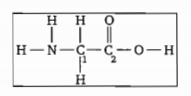When the substances Si, KCl, $C{H}_{3}$OH and ${C}_{2}{H}_{6}$ are arranged in order of increasing melting point , what is the correct order?

(a) Si, KCl ,$C{H}_{3}$OH ,${C}_{2}{H}_{6}$

(b) $C{H}_{3}OH$${C}_{2}{H}_{6}$, Si ,KCl

(c) KCl, Si, ${C}_{2}{H}_{6}$$C{H}_{3}OH$

(d)

Concept Questions :-

Lattice/Hydration Energy
High Yielding Test Series + Question Bank - NEET 2020

Difficulty Level:

Iodine molecules are held in the solid lattice by............

(a) London forces                     (b) dipole-dipole interactions

(c) Covalent bonds                   (d) coulombic force

Concept Questions :-

Vanderwaal Force
High Yielding Test Series + Question Bank - NEET 2020

Difficulty Level:

Which substance has the highest melting point ?

(a) CO

(b) $C{O}_{2}$

(d) $Si{O}_{2}$

(d) ${P}_{2}{O}_{5}$

Concept Questions :-

Lattice/Hydration Energy
High Yielding Test Series + Question Bank - NEET 2020

Difficulty Level:

Nodal planes of

(a) all are in molecular plane

(b) two in a molecular plane and in a plane peerpendicular to moleclar plane which contains

(c) one in molecular plane and two in plane perpendicular to molecular plane which bisects

(d) two in a molecular plane and one in a plane perpendicular to molecular plane which bisects  at right angle.

Concept Questions :-

Hybridisation
High Yielding Test Series + Question Bank - NEET 2020

Difficulty Level:

The hybridisation of the  central  atom will change when:

(a) $N{H}_{3}$ combines with ${H}^{+}$

(b) ${H}_{3}B{O}_{{3}_{}}$combines with $O{H}^{-}$

(c) $N{H}_{3}$ forms $N{{H}_{2}}^{-}$

(d) combines with ${H}^{+}$

Concept Questions :-

Hybridisation
High Yielding Test Series + Question Bank - NEET 2020

Difficulty Level:

Spacies having maximum $\text{'}CI—O\text{'}$ bond order is:

Concept Questions :-

Polarity
High Yielding Test Series + Question Bank - NEET 2020

Difficulty Level:

Which of the following have $\mathrm{X}-\mathrm{O}-\mathrm{X}$ linkage?

(where X is Central atom)

(i) $C{r}_{2}{O}_{7}^{2-}$                            (ii) ${\mathrm{S}}_{2}{\mathrm{O}}_{3}^{2-}$

(iii) Pyrosilicate                     (iv) Hyponitrous Acid

(a) (i) (iii)                                             (b) (iii) (iv)

(c) (i) (iii) (iv)                                       (d) (i) (ii)

Concept Questions :-

Types of Bonding
High Yielding Test Series + Question Bank - NEET 2020

Difficulty Level:

Which bonds are formed by a carbon atom with $s{p}^{2}$ - hybridisation?

(a)

(b)

(c)

(d)

Concept Questions :-

Hybridisation
High Yielding Test Series + Question Bank - NEET 2020

Difficulty Level:

Which of the following species contains maximum number of atoms in 'XY' plane?

Concept Questions :-

Hybridisation
High Yielding Test Series + Question Bank - NEET 2020

Difficulty Level:

What are the hybridisation of the carbon atoms labelled ${C}_{1}$ and ${C}_{2}$ respectively in glycein?${C}_{1}$             ${C}_{2}$

(a) $s{p}^{2}$         (a) $s{p}^{2}$

(b) $s{p}^{2}$         (b) $s{p}^{3}$

(c) $s{p}^{3}$          (c) $s{p}^{2}$

(d) $sp$          (d) s${p}^{3}$

Concept Questions :-

Hybridisation